L* K1-086直线电机模组 L*L1-112直线电机模组 L*M1-140直线电机模组 L*S1-140直线电机模组 L1S2-140直线电机模组 L1F1-170直线电机模组 L1F2-170直线电机模组 L1G1-210直线电机模组 L1G2-210直线电机模组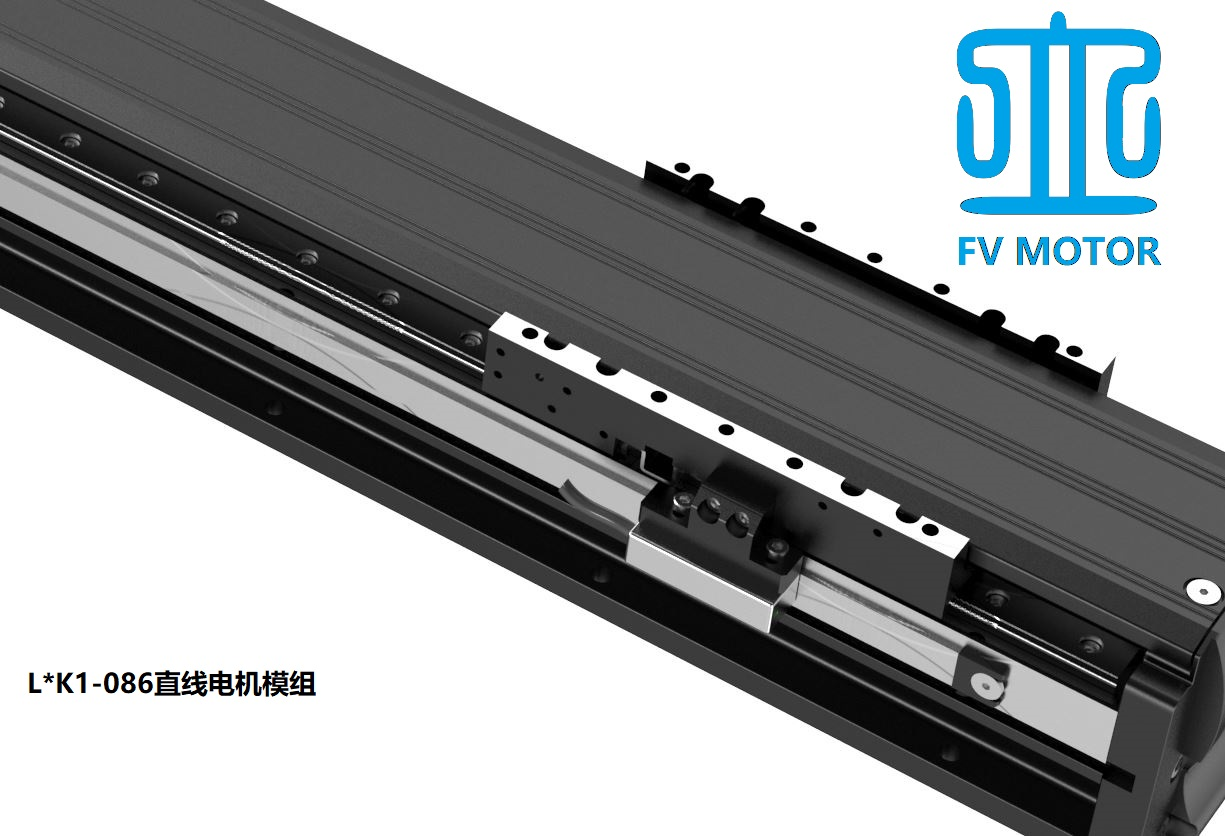1、连续推力105N，峰值推力240N   2、底座宽度86mm，安装高度81mm3、标配SRS12M型THK双导轨4、引接线300mm（可订制，具体咨询伏为电机单独办事处或经销商） 5、标配磁栅尺反馈，重复定位精度±5微米            （可选配光栅尺反馈，精度±2微米）6、最高速度运行速度3000mm/s7、最佳使用工况：                加速度：    1g                    驻留时间：    200ms                安装方式：    水平安装                速度1m/s时负载不超过10kg                速度2m/s时负载不超过5kg 有效行程 模组总长 单动子型号 双动子型号 L=042 L=252 L1K1-086M042-R-B L=106 L=316 L1K1-086M106-R-B L=170 L=380 L1K1-086M170-R-B L=234 L=444 L1K1-086M234-R-B L=298 L=508 L1K1-086M298-R-B L=362 L=572 L1K1-086M362-R-B L2K1-086M362-R-B L=426 L=636 L1K1-086M426-R-B L2K1-086M426-R-B L=490 L=700 L1K1-086M490-R-B L2K1-086M490-R-B L=554 L=764 L1K1-086M554-R-B L2K1-086M554-R-B L=618 L=828 L1K1-086M618-R-B L2K1-086M618-R-B L=682 L=892 L1K1-086M682-R-B L2K1-086M682-R-B L=746 L=956 L1K1-086M746-R-B L2K1-086M746-R-B L=810 L=1020 L1K1-086M810-R-B L2K1-086M810-R-B L=874 L=1084 L1K1-086M874-R-B L2K1-086M874-R-B L=938 L=1148 L1K1-086M938-R-B L2K1-086M938-R-B L=1002 L=1212 L1K1-086M1002-R-B L2K1-086M1002-R-B L=1066 L=1276 L1K1-086M1066-R-B L2K1-086M1066-R-B L=1130 L=1340 L1K1-086M1130-R-B L2K1-086M1130-R-B L=1194 L=1404 L1K1-086M1194-R-B L2K1-086M1194-R-B L=1258 L=1468 L1K1-086M1258-R-B L2K1-085M1258-R-B L=1322 L=1532 L1K1-086M1322-R-B L2K1-086M1322-R-B L=1386 L=1596 L1K1-086M1386-R-B L2K1-086M1386-R-B L=1450 L=1660 L1K1-086M1450-R-B L2K1-086M1450-R-B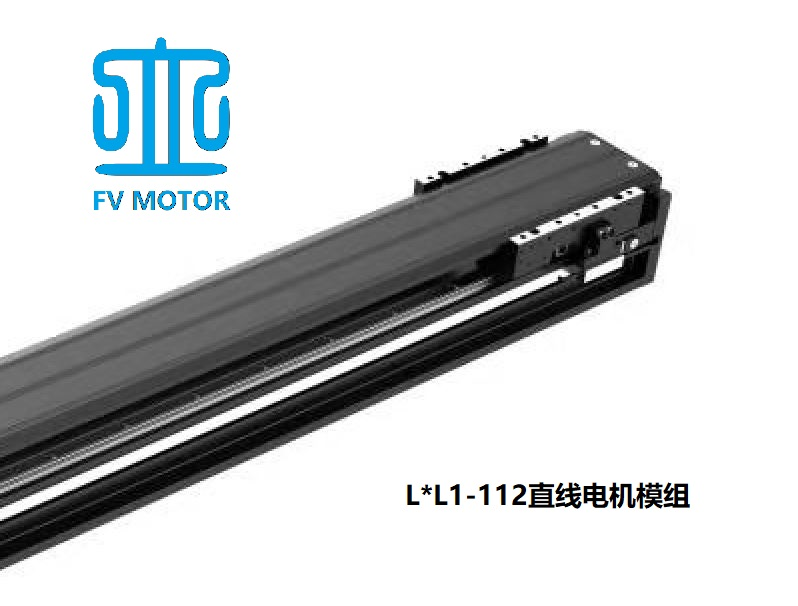1、连续推力200N，峰值推力500N2、底座宽度112mm，安装高度94mm　3、标配SSR15XV型THK双导轨4、引接线300mm（可订制，具体咨询伏为电机单独办事处或经销商）5、标配磁栅尺反馈，重复定位精度±5微米            （可选配光栅尺反馈，精度±2微米）6、最高速度运行速度3000mm/s7、最佳使用工况：            　加速度：    1g                驻留时间：    200ms               安装方式：    水平安装               速度1m/s时负载不超过20kg               速度2m/s时负载不超过8kg 有效行程 模组总长 单动子型号 双动子型号 L=042 L=252 L1L1-112M042-R-B L=106 L=316 L1L1-112M106-R-B L=170 L=380 L1L1-112M170-R-B L=234 L=444 L1L1-112M234-R-B L=298 L=508 L1L1-112M298-R-B L=362 L=572 L1L1-112M362-R-B L2L1-112M362-R-B L=426 L=636 L1L1-112M426-R-B L2L1-112M426-R-B L=490 L=700 L1L1-112M490-R-B L2L1-112M490-R-B L=554 L=764 L1L1-112M554-R-B L2L1-112M554-R-B L=618 L=828 L1L1-112M618-R-B L2L1-112M618-R-B L=682 L=892 L1L1-112M682-R-B L2L1-112M682-R-B L=746 L=956 L1L1-112M746-R-B L2L1-112M746-R-B L=810 L=1020 L1L1-112M810-R-B L2L1-112M810-R-B L=874 L=1084 L1L1-112M874-R-B L2L1-112M874-R-B L=938 L=1148 L1L1-112M938-R-B L2L1-112M938-R-B L=1002 L=1212 L1L1-112M1002-R-B L2L1-112M1002-R-B L=1066 L=1276 L1L1-112M1066-R-B L2L1-112M1066-R-B L=1130 L=1340 L1L1-112M1130-R-B L2L1-112M1130-R-B L=1194 L=1404 L1L1-112M1194-R-B L2L1-112M1194-R-B L=1258 L=1468 L1L1-112M1258-R-B L2L1-112M1258-R-B L=1322 L=1532 L1L1-112M1322-R-B L2L1-112M1322-R-B L=1386 L=1596 L1L1-112M1386-R-B L2L1-112M1386-R-B L=1450 L=1660 L1L1-112M1450-R-B L2L1-112M1450-R-B L=1514 L=1724 L1L1-112M1514-R-B L2L1-112M1514-R-B L=1578 L=1788 L1L1-1120M1578-R-B L2L1-112M1578-R-B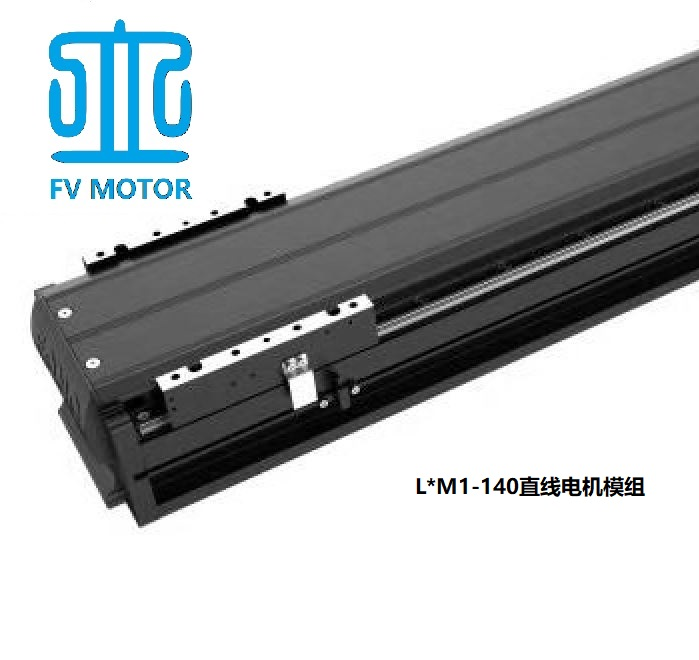1、连续推力315N，峰值推力635N2、底座宽度140mm，安装高度97mm　3、标配SSR15XV型THK双导轨4、引接线300mm（可订制，具体咨询伏为电机单独办事处或经销商）5、标配磁栅尺反馈，重复定位精度±5微米            （可选配光栅尺反馈，精度±2微米）6、最高速度运行速度3000mm/s7、最佳使用工况：            　加速度：    1g               驻留时间：    200ms               安装方式：    水平安装               速度1m/s时负载不超过35kg               速度2m/s时负载不超过15kg 有效行程 模组总长 单动子型号 双动子型号 L=042 L=260 L1M1-140M042-R-B L=106 L=324 L1M1-140M106-R-B L=170 L=388 L1M1-140M170-R-B L=234 L=452 L1M1-140M234-R-B L=298 L=516 L1M1-140M298-R-B L=362 L=580 L1M1-140M362-R-B L2M1-140M362-R-B L=426 L=644 L1M1-140M426-R-B L2M1-140M426-R-B L=490 L=708 L1M1-140M490-R-B L2M1-140M490-R-B L=554 L=772 L1M1-140M554-R-B L2M1-140M554-R-B L=618 L=836 L1M1-140M618-R-B L2M1-140M618-R-B L=682 L=900 L1M1-140M682-R-B L2M1-140M682-R-B L=746 L=964 L1M1-140M746-R-B L2M1-140M746-R-B L=810 L=1028 L1M1-140M810-R-B L2M1-140M810-R-B L=874 L=1092 L1M1-140M874-R-B L2M1-140M874-R-B L=938 L=1156 L1M1-140M938-R-B L2M1-140M938-R-B L=1002 L=1220 L1M1-140M1002-R-B L2M1-140M1002-R-B L=1066 L=1284 L1M1-140M1066-R-B L2M1-140M1066-R-B L=1130 L=1348 L1M1-140M1130-R-B L2M1-140M1130-R-B L=1194 L=1412 L1M1-140M1194-R-B L2M1-140M1194-R-B L=1258 L=1476 L1M1-140M1258-R-B L2M1-140M1258-R-B L=1322 L=1540 L1M1-140M1322-R-B L2M1-140M1322-R-B L=1386 L=1604 L1M1-140M1386-R-B L2M1-140M1386-R-B L=1450 L=1668 L1M1-140M1450-R-B L2M1-140M1450-R-B L=1514 L=1732 L1M1-140M1514-R-B L2M1-140M1514-R-B L=1578 L=1796 L1M1-140M1578-R-B L2M1-140M1578-R-B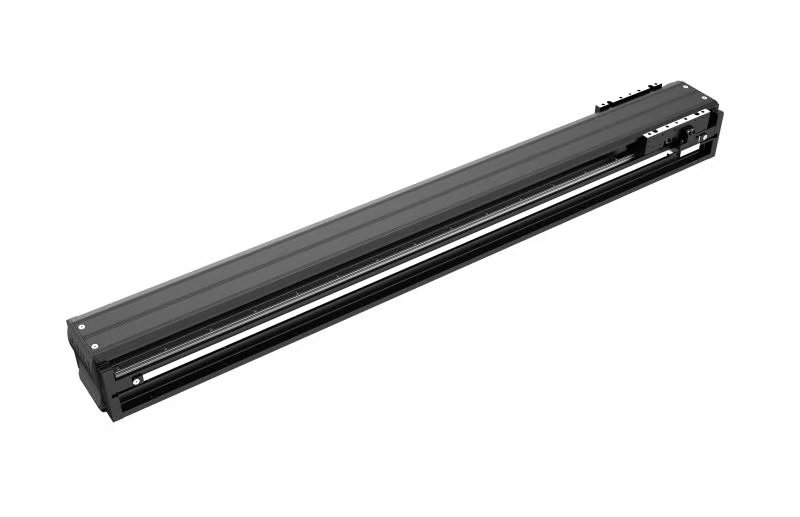1、连续推力200N，峰值推力390N

2、底座宽度140mm，安装高度79mm

3、标配SSR15型THK双导轨

4、引接线300mm（可订制，具体咨询伏为电机单独办事处或经销商）

5、标配磁栅尺反馈，重复定位精度±5微米（可选配光栅尺反馈，精度更高）

6、最高速度运行速度3000mm/s

7、最佳使用工况：

加速度：    1g

驻留时间：    200ms

安装方式：    水平安装

速度1m/s时负载不超过20kg

速度2m/s时负载不超过8kg

 有效行程 模组总长 单动子型号 L=106 L=314 L1S1-140M106-R-B L=170 L=378 L1S1-140M170-R-B L=234 L=442 L1S1-140M234-R-B L=298 L=506 L1S1-140M298-R-B L=362 L=570 L1S1-140M362-R-B L=426 L=634 L1S1-140M426-R-B L=490 L=698 L1S1-140M490-R-B L=554 L=762 L1S1-140M554-R-B L=618 L=826 L1S1-140M618-R-B L=682 L=890 L1S1-140M682-R-B L=746 L=954 L1S1-140M746-R-B L=810 L=1018 L1S1-140M810-R-B L=874 L=1082 L1S1-140M874-R-B L=938 L=1146 L1S1-140M938-R-B L=1002 L=1210 L1S1-140M1002-R-B L=1066 L=1274 L1S1-140M1066-R-B L=1130 L=1338 L1S1-140M1130-R-B L=1194 L=1402 L1S1-140M1194-R-B L=1258 L=1466 L1S1-140M1258-R-B L=1322 L=1530 L1S1-140M1322-R-B L=1386 L=1594 L1S1-140M1386-R-B L=1450 L=1658 L1S1-140M1450-R-B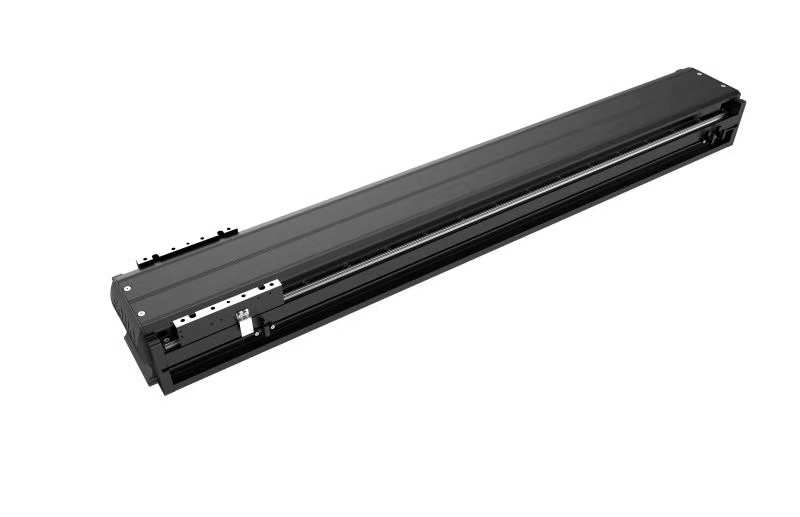1、连续推力320N，峰值推力608N

2、底座宽度140mm，安装高度79mm

3、标配SSR15型THK双导轨

4、引接线300mm（可订制，具体咨询伏为电机单独办事处或经销商）

5、标配磁栅尺反馈，重复定位精度±5微米（可选配光栅尺反馈，精度更高）

6、最高速度运行速度3000mm/s

7、最佳使用工况：

加速度：    1g

驻留时间：    200ms

安装方式：    水平安装

速度1m/s时负载不超过35kg

速度2m/s时负载不超过15kg

 有效行程 模组总长 单动子型号 L=122 L=442 L1S2-140M106-R-B L=186 L=506 L1S2-140M170-R-B L=250 L=570 L1S2-140M250-R-B L=314 L=634 L1S2-140M314-R-B L=378 L=698 L1S2-140M378-R-B L=442 L=762 L1S2-140M442-R-B L=506 L=826 L1S2-140M506-R-B L=570 L=890 L1S2-140M570-R-B L=634 L=954 L1S2-140M634-R-B L=698 L=1018 L1S2-140M698-R-B L=762 L=1082 L1S2-140M762-R-B L=826 L=1146 L1S2-140M826-R-B L=890 L=1210 L1S2-140M890-R-B L=954 L=1274 L1S2-140M954-R-B L=1018 L=1338 L1S2-140M1018-R-B L=1082 L=1402 L1S2-140M1082-R-B L=1146 L=1466 L1S2-140M1146-R-B L=1210 L=1530 L1S2-140M1210-R-B L=1274 L=1594 L1S2-140M1274-R-B L=1338 L=1658 L1S2-140M1338-R-B L=1402 L=1722 L1S2-140M1402-R-B L=1466 L=1786 L1S2-140M1466-R-B L=1530 L=1850 L1S2-140M1530-R-B L=1594 L=1914 L1S2-140M1594-R-B L=1658 L=1978 L1S2-140M1658-R-B L=1722 L=2042 L1S2-140M1722-R-B L=1786 L=2106 L1S2-140M1786-R-B L=1850 L=2170 L1S2-140M1850-R-B L=1914 L=2234 L1S2-140M1914-R-B L=1978 L=2298 L1S2-140M1978-R-B L=2042 L=2362 L1S2-140M2042-R-B1、连续推力310N，峰值推力589N

2、底座宽度170mm，安装高度91mm

3、标配SSR15型THK双导轨

4、引接线300mm（可订制，具体咨询伏为电机单独办事处或经销商）

5、标配磁栅尺反馈，重复定位精度±5微米（可选配光栅尺反馈，精度更高）

6、最高速度运行速度3000mm/s

7、最佳使用工况：

加速度：    1g

驻留时间：    200ms

安装方式：    水平安装

速度1m/s时负载不超过35kg

速度2m/s时负载不超过15kg

 有效行程 模组总长 单动子型号 L=106 L=314 L1F1-170M106-R-B L=170 L=378 L1F1-170M170-R-B L=234 L=442 L1F1-170M234-R-B L=298 L=506 L1F1-170M298-R-B L=362 L=570 L1F1-170M362-R-B L=426 L=634 L1F1-170M426-R-B L=490 L=698 L1F1-170M490-R-B L=554 L=762 L1F1-170M554-R-B L=618 L=826 L1F1-170M618-R-B L=682 L=890 L1F1-170M682-R-B L=746 L=954 L1F1-170M746-R-B L=810 L=1018 L1F1-170M810-R-B L=874 L=1082 L1F1-170M874-R-B L=938 L=1146 L1F1-170M938-R-B L=1002 L=1210 L1F1-170M1002-R-B L=1066 L=1274 L1F1-170M1066-R-B L=1130 L=1338 L1F1-170M1130-R-B L=1194 L=1402 L1F1-170M1194-R-B L=1258 L=1466 L1F1-170M1258-R-B L=1322 L=1530 L1F1-170M1322-R-B L=1386 L=1594 L1F1-170M1386-R-B L=1450 L=1658 L1F1-170M1450-R-B L=1514 L=1722 L1F1-170M1514-R-B L=1578 L=1786 L1F1-170M1578-R-B L=1642 L=1850 L1F1-170M1642-R-B L=1706 L=1914 L1F1-170M1706-R-B L=1770 L=1978 L1F1-170M1770-R-B L=1834 L=2042 L1F1-170M1834-R-B L=1898 L=2106 L1F1-170M1898-R-B L=1962 L=2170 L1F1-170M1962-R-B L=2026 L=2234 L1F1-170M2026-R-B1、连续推力550N，峰值推力1045N

2、底座宽度170mm，安装高度91mm

3、标配SSR15型THK双导轨

4、引接线300mm（可订制，具体咨询伏为电机单独办事处或经销商）

5、标配磁栅尺反馈，重复定位精度±5微米（可选配光栅尺反馈，精度更高）

6、最高速度运行速度3000mm/s

7、最佳使用工况：

加速度：    1g

驻留时间：    200ms

安装方式：    水平安装

速度1m/s时负载不超过50kg

速度2m/s时负载不超过25kg

 有效行程 模组总长 单动子型号 L=122 L=442 L1F2-170M122-R-B L=186 L=506 L1F2-170M186-R-B L=250 L=570 L1F2-170M250-R-B L=314 L=634 L1F2-170M314-R-B L=378 L=698 L1F2-170M378-R-B L=442 L=762 L1F2-170M442-R-B L=506 L=826 L1F2-170M506-R-B L=570 L=890 L1F2-170M570-R-B L=634 L=954 L1F2-170M634-R-B L=698 L=1018 L1F2-170M698-R-B L=762 L=1082 L1F2-170M762-R-B L=826 L=1146 L1F2-170M826-R-B L=890 L=1210 L1F2-170M890-R-B L=954 L=1274 L1F2-170M954-R-B L=1018 L=1338 L1F2-170M1018-R-B L=1082 L=1402 L1F2-170M1082-R-B L=1146 L=1466 L1F2-170M1146-R-B L=1210 L=1530 L1F2-170M1210-R-B L=1274 L=1594 L1F2-170M1274-R-B L=1338 L=1658 L1F2-170M1338-R-B L=1402 L=1722 L1F2-170M1402-R-B L=1466 L=1786 L1F2-170M1466-R-B L=1530 L=1850 L1F2-170M1530-R-B L=1594 L=1914 L1F2-170M1594-R-B L=1658 L=1978 L1F2-170M1658-R-B L=1722 L=2042 L1F2-170M1722-R-B L=1786 L=2106 L1F2-170M1786-R-B L=1850 L=2170 L1F2-170M1850-R-B L=1914 L=2234 L1F2-170M1914-R-B L=1978 L=2298 L1F2-170M1978-R-B L=2042 L=2362 L1F2-170M2042-R-B1、连续推力420N，峰值推力798N

2、底座宽度210mm，安装高度99.5mm

3、标配SSR20型THK双导轨

4、引接线300mm（可订制，具体咨询伏为电机单独办事处或经销商）

5、标配磁栅尺反馈，重复定位精度±5微米（可选配光栅尺反馈，精度更高）

6、最高速度运行速度3000mm/s

7、最佳使用工况：

加速度：    1g

驻留时间：    200ms

安装方式：    水平安装

速度1m/s时负载不超过40kg

速度2m/s时负载不超过20kg

 有效行程 模组总长 单动子型号 L=106 L=314 L1G1-210M106-R-B L=170 L=378 L1G1-210M170-R-B L=234 L=442 L1G1-210M234-R-B L=298 L=506 L1G1-210M298-R-B L=362 L=570 L1G1-210M362-R-B L=426 L=634 L1G1-210M426-R-B L=490 L=698 L1G1-210M490-R-B L=554 L=762 L1G1-210M554-R-B L=618 L=826 L1G1-210M618-R-B L=682 L=890 L1G1-210M682-R-B L=746 L=954 L1G1-210M746-R-B L=810 L=1018 L1G1-210M810-R-B L=874 L=1082 L1G1-210M874-R-B L=938 L=1146 L1G1-210M938-R-B L=1002 L=1210 L1G1-210M1002-R-B L=1066 L=1274 L1G1-210M1066-R-B L=1130 L=1338 L1G1-210M1130-R-B L=1194 L=1402 L1G1-210M1194-R-B L=1258 L=1466 L1G1-210M1258-R-B L=1322 L=1530 L1G1-210M1322-R-B L=1386 L=1594 L1G1-210M1386-R-B L=1450 L=1658 L1G1-210M1450-R-B L=1514 L=1722 L1G1-210M1514-R-B L=1578 L=1786 L1G1-210M1578-R-B L=1642 L=1850 L1G1-210M1642-R-B L=1706 L=1914 L1G1-210M1706-R-B L=1770 L=1978 L1G1-210M1770-R-B L=1834 L=2042 L1G1-210M1834-R-B L=1898 L=2106 L1G1-210M1898-R-B L=1962 L=2170 L1G1-210M1962-R-B L=2026 L=2234 L1G1-210M2026-R-B1、连续推力710N，峰值推力1349N

2、底座宽度210mm，安装高度99.5mm

3、标配SSR20型THK双导轨

4、引接线300mm（可订制，具体咨询伏为电机单独办事处或经销商）

5、标配磁栅尺反馈，重复定位精度±5微米（可选配光栅尺反馈，精度更高）

6、最高速度运行速度3000mm/s

7、最佳使用工况：

加速度：    1g

驻留时间：    200ms

安装方式：    水平安装

速度1m/s时负载不超过60kg

速度2m/s时负载不超过35kg

 有效行程 模组总长 单动子型号 L=122 L=442 L1G2-210M122-R-B L=186 L=506 L1G2-210M186-R-B L=250 L=570 L1G2-210M250-R-B L=314 L=634 L1G2-210M314-R-B L=378 L=698 L1G2-210M378-R-B L=442 L=762 L1G2-210M442-R-B L=506 L=826 L1G2-210M506-R-B L=570 L=890 L1G2-210M570-R-B L=634 L=954 L1G2-210M634-R-B L=698 L=1018 L1G2-210M698-R-B L=762 L=1082 L1G2-210M762-R-B L=826 L=1146 L1G2-210M826-R-B L=890 L=1210 L1G2-210M890-R-B L=954 L=1274 L1G2-210M954-R-B L=1018 L=1338 L1G2-210M1018-R-B L=1082 L=1402 L1G2-210M1082-R-B L=1146 L=1466 L1G2-210M1146-R-B L=1210 L=1530 L1G2-210M1210-R-B L=1274 L=1594 L1G2-210M1274-R-B L=1338 L=1658 L1G2-210M1338-R-B L=1402 L=1722 L1G2-210M1402-R-B L=1466 L=1786 L1G2-210M1466-R-B L=1530 L=1850 L1G2-210M1530-R-B L=1594 L=1914 L1G2-210M1594-R-B L=1658 L=1978 L1G2-210M1658-R-B L=1722 L=2042 L1G2-210M1722-R-B L=1786 L=2106 L1G2-210M1786-R-B L=1850 L=2170 L1G2-210M1850-R-B L=1914 L=2234 L1G2-210M1914-R-B L=1978 L=2298 L1G2-210M1978-R-B L=2042 L=2362 L1G2-210M2042-R-B